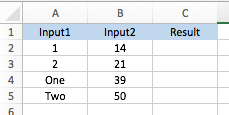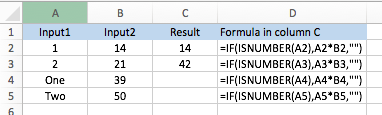# How to check if a cell contains a valid number

When writing a formula that references other cells, it can sometimes be useful to check that those cells contain a valid value. In this lesson, we'll look at Excel's ISNUMBER function as a way of doing this.

I was recently asked by a reader to provide a formula that would return the result of a calculation if a certain value cell contained a number, and leave the cell blank if that value cell contained anything that wasn't a valid number.

There are various ways to check for errors in an Excel formula. In this example, the best function to use is the ISNUMBER function.

## ISNUMBER Syntax

ISNUMBER has a very simple syntax:

=ISNUMBER(value)

The ISNUMBER function will return either TRUE or FALSE. For the purposes of writing additional formulas, you can consider TRUE = 1 and FALSE = 0. I'll show you an example later in this lesson.

The value you provide to ISNUMBER can be a reference to another cell, such as:

• =ISNUMBER(A1)

It can also be a direct value:

• =ISNUMBER(3) returns TRUE

Here are some examples:

• =ISNUMBER(1)  returns TRUE
• =ISNUMBER("1") returns FALSE
• =ISNUMBER(five) returns FALSE
• =ISNUMBER(3) * 30 returns 30 (remember that TRUE = 1, so TRUE * 30 is the same as 1 * 30)
• =ISNUMBER(happy) * 30 returns 0 (remember that FALSE = 0, so FALSE * 30 is the same as 0 * 30)

## A worked example for the ISNUMBER function.

As I mentioned earlier, this lesson was inspired by someone who asked how to return the results of a calculation if a certain cell contained a number, and leave the cell blank if the cell did not contain a number. This scenario is preferable to seeing #VALUE! if the cell doesn't contain a number.

In this case, I'll use Excel's IF function combined with the ISNUMBER function to get the right outcome.

Suppose we have this example (it's a simple calculation simply to illustrate the point).We need to calculate the Result in column C, but only when column A contains a valid number. Here is the formula to use in C2:

• =IF(ISNUMBER(A2),A2*B2,"")

Here's what this formula does:

• First, it checks the contents of A2.
• If A2 contains a number, the formula calculates A2*B2 and returns the result.
• If A2 doesn't contain a number, the formula simply returns "" - quote marks with nothing between them. In doing so, cell C2 appears to be empty.

Note that you could, if  you prefer, put something between the quote marks to warn other users that there is an invalid value in A2. For example:

• =IF(ISNUMBER(A2),A2*B2,"Not valid")

Here's the finished version of our example:Note that although C4 and C5 appear to be empty, they are not - they still contain our formula. Changing the values in A4 or A5 would change the values in those cells so they are no longer blank.

## Summary

The ISNUMBER function is a quick, easy way to check if a cell contains a number. Our example used the IF function to illustrate how ISNUMBER can be used. If you haven't used IF in a formula before, you can read our lesson on the IF function here.